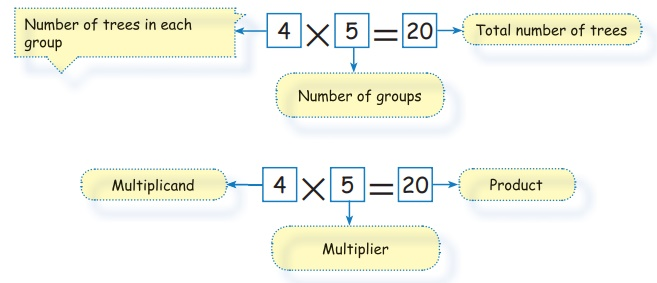Home | | Maths 3rd Std | Symbol of multiplication

# Symbol of multiplication

we use the symbol “×” to represent multiplication.

Symbol of multiplication

we use the symbol “×” to represent multiplication.4 Trees in 5 groups is 20

This can be written as 4 × 5 = 20Mutiplication of a number with other number can be done in the following ways.

(i) Dot multiplication

(iii) Regrouping

(iv) Standard multiplication algorithm

(v) Lattice multiplication

Tags : Numbers | Term 2 Chapter 1 | 3rd Maths , 3rd Maths : Term 2 Unit 1 : Numbers
Study Material, Lecturing Notes, Assignment, Reference, Wiki description explanation, brief detail
3rd Maths : Term 2 Unit 1 : Numbers : Symbol of multiplication | Numbers | Term 2 Chapter 1 | 3rd Maths